# Understanding & Implementing XGBoost - A Machine Learning Algorithm In Data ScienceRegardless of XGBoost having a unique name, it is actually not hard to understand as long as we go through a few concepts around it. Terms like decision trees, learning algorithms, gradient boosting, and a few more, may sound futuristic. Let’s walk you through the basics of XGBoost and a few of the related terms and then we’ll understand the process of implementing it in data science.

## What is XGBoost?

XGBoost is an open-source library that follows the optimized distributed gradient boosting methodology in machine learning models and learning algorithms. It offers a gradient boosting framework. XGBoost stands for extreme gradient boosting and is a distributed gradient boosted decision tree (GBDT). Implementing XGBoost paves way for parallel tree boosting and is one of the popular machine learning libraries for classification, ranking, and regression.

Apart from that, XGBoost is one of the leading techniques used in Kaggle competitions because of its prediction ability and also because it is developer friendly.

It is important to understand the algorithms and machine learning concepts that XGBoost deals with. These are a few areas where implementing XGBoost saves time and improves the code efficiency.

• Decision trees

• Supervised machine learning

• Ensemble learning

Let’s walk you through these aspects in detail.

Gradient boosting is widely used in machine learning algorithms. Errors and anomalies in machine learning algorithms are classified as:

• Variance error

• Bias error

Gradient boosting, as the name suggests, is a boosting algorithm. It eliminates the errors and improves the performance of the model.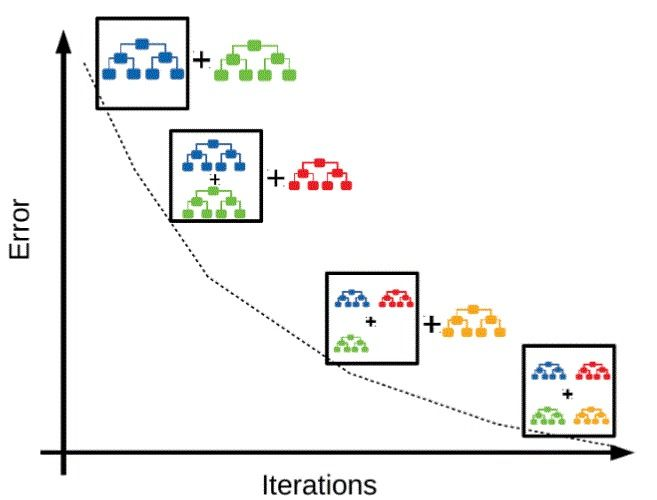Image source

Apart from that, gradient boosting algorithm can be used to predict a categorical target as a classifier and continuous target as a regressor. When it is used as a classifier, the cost function is log loss. And when it is used as a regressor, the cost function is Mean Square Error.

### Decision trees

A decision tree is a type of supervised machine learning model wherein the data is continuously split according to the conditions. It is used as a tree-like model for multiple decisions. These trees can be used to map out an algorithm that predicts the best choice and can also be used to drive informal discussions.

A decision tree starts with a single code that further branches into possible outcomes. Each outcome leads to additional nodes that branch into more possibilities. This entire procedure gives it a tree-like shape and it is similar to a controlled fission reaction.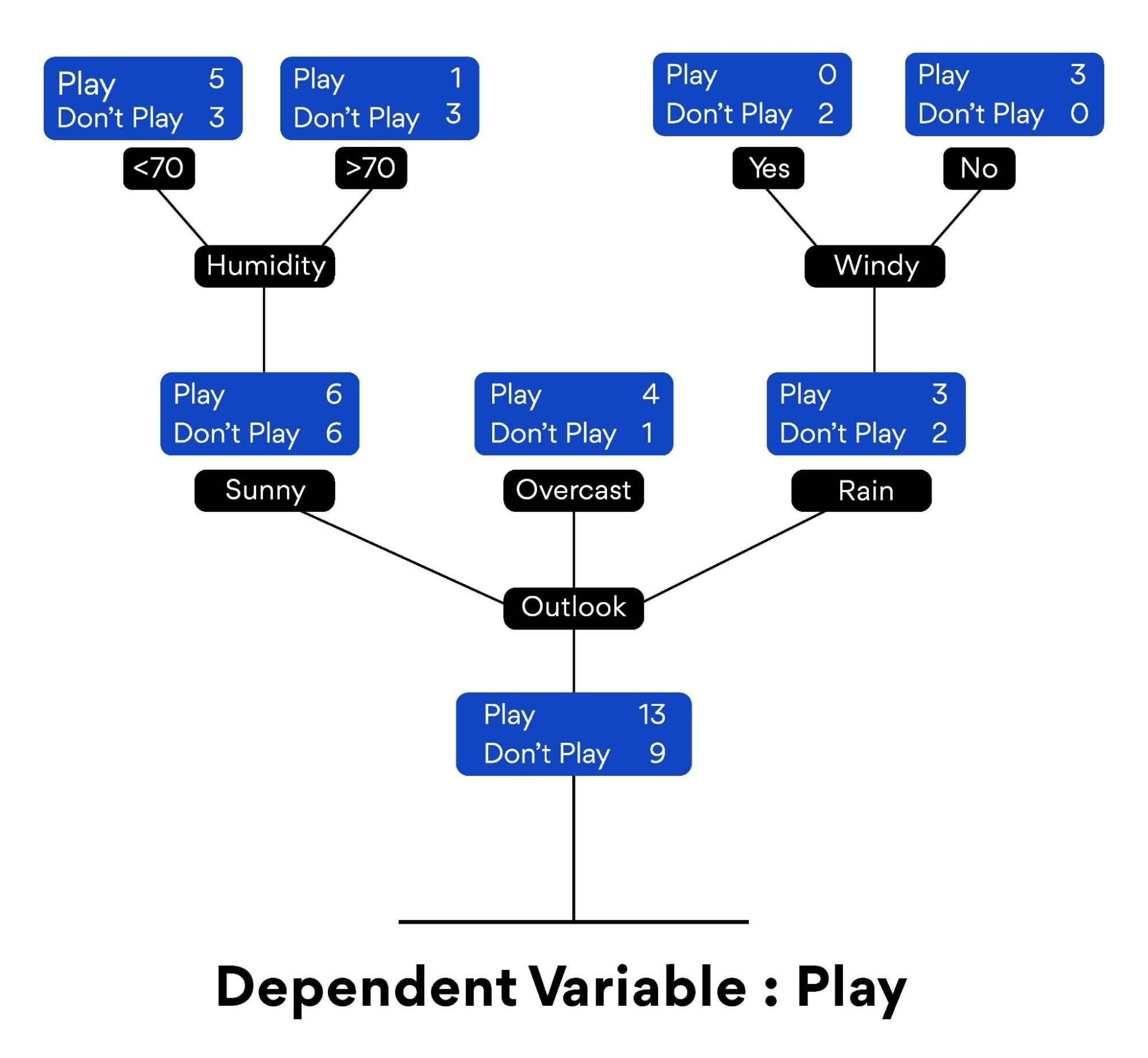There are different types of nodes.

• Decision nodes

• Chance nodes

• End nodes

These nodes are represented by a circle that shows the possibilities of certain outcomes. A decision node is represented by a square and portrays a decision that needs to be made. An end node shows the final outcome of a decision path.

### Supervised machine learning

Supervised learning is a type of machine learning where trained data is used to predict the output. In this type of machine learning, the training data is provided to the machines as the supervisor works and the machine is given all the necessary information to predict an error-free output.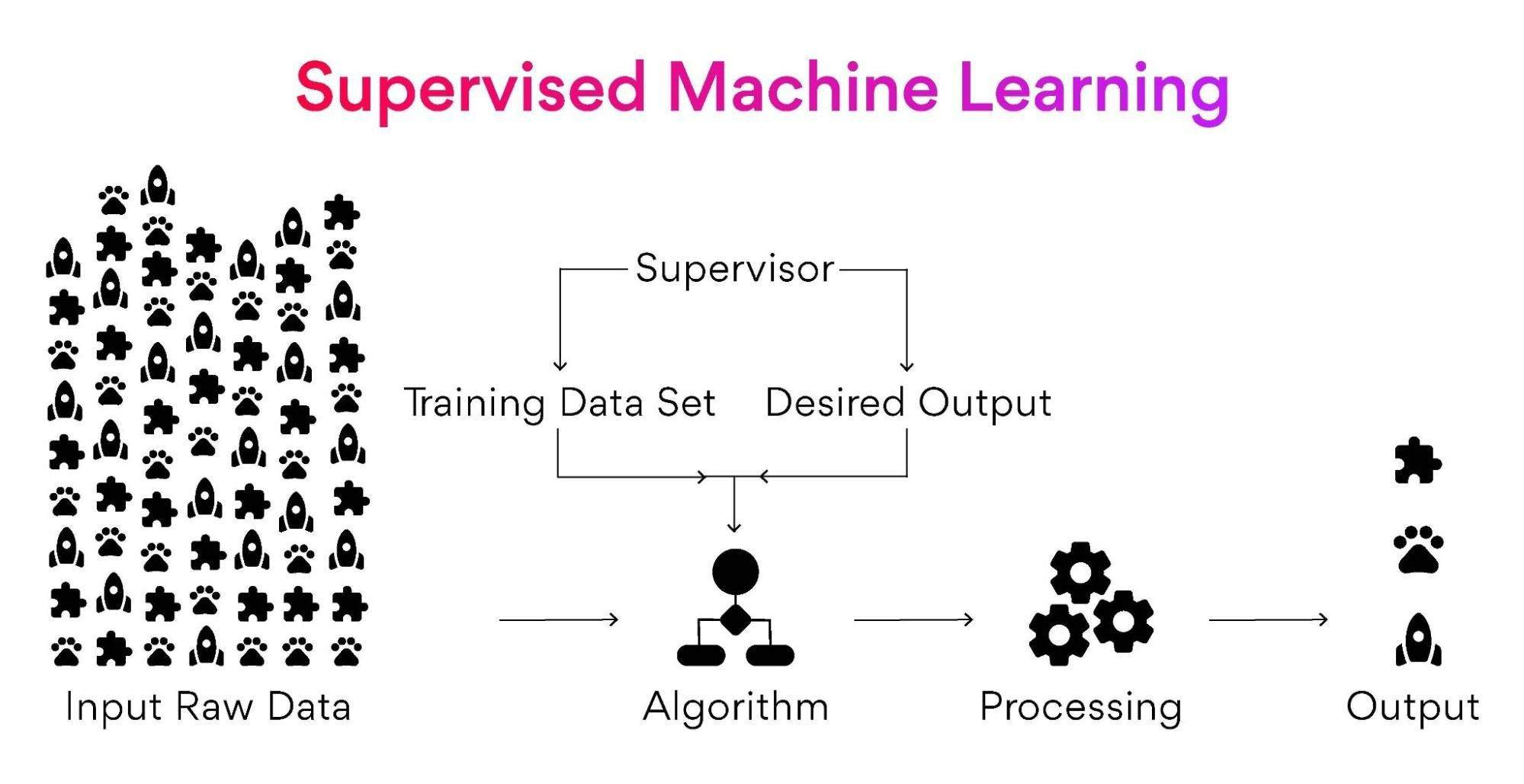The main aim behind this type of machine learning is to find a mapping function to map the input variable (x) with that of the output variable (y).

### Ensemble learning

Ensemble learning is a process by which multiple models including classifiers and regressors are strategically created and combined to solve a computational problem. This type of learning is used to improve the parameters of model performance such as prediction, function approximation, and classification.

Ensemble learning is a meta approach to machine learning for better predictive model performance. It combines the predictions from multiple models for better outcomes.

There are three main classes of ensemble learning:

1. Bagging

Bagging is a class that fits several decision trees of the same data sets on different samples. It also streamlines the prediction averages.

2. Stacking

Stacking deals with fitting several different learning models on the same data while using another learning model to combine the predictions in the best way possible.

3. Boosting

Boosting adds ensemble members that correct the predictions made by prior machine learning models. The main aim of this class is to analyze the output and correct the predictions.

## XGBoost functionality

After having an idea about gradient boosting, machine learning models, decision trees, and learning algorithms, implementing XGBoost will be easy to understand. XGBoost was designed for optimal speed and model performance. In this, a hyper-parameter is set before the learning process begins and directly affects the model’s performance.

### What is a hyperparameter?

A hyperparameter is a value that determines the learning process of a learning algorithm. XGBoost offers a wide range of hyperparameters. We can easily leverage the complete potential of XGBoost by tuning its hyperparameters.

This leads to a question: What are the important hyperparameters and which one to choose when implementing XGBoost? How to use these hyperparameters?

Here are a few Hyperparameters associated with XGBoost.

• Booster

• reg_alpha and reg_lambda

• max_depth

• subsample

• num_estimators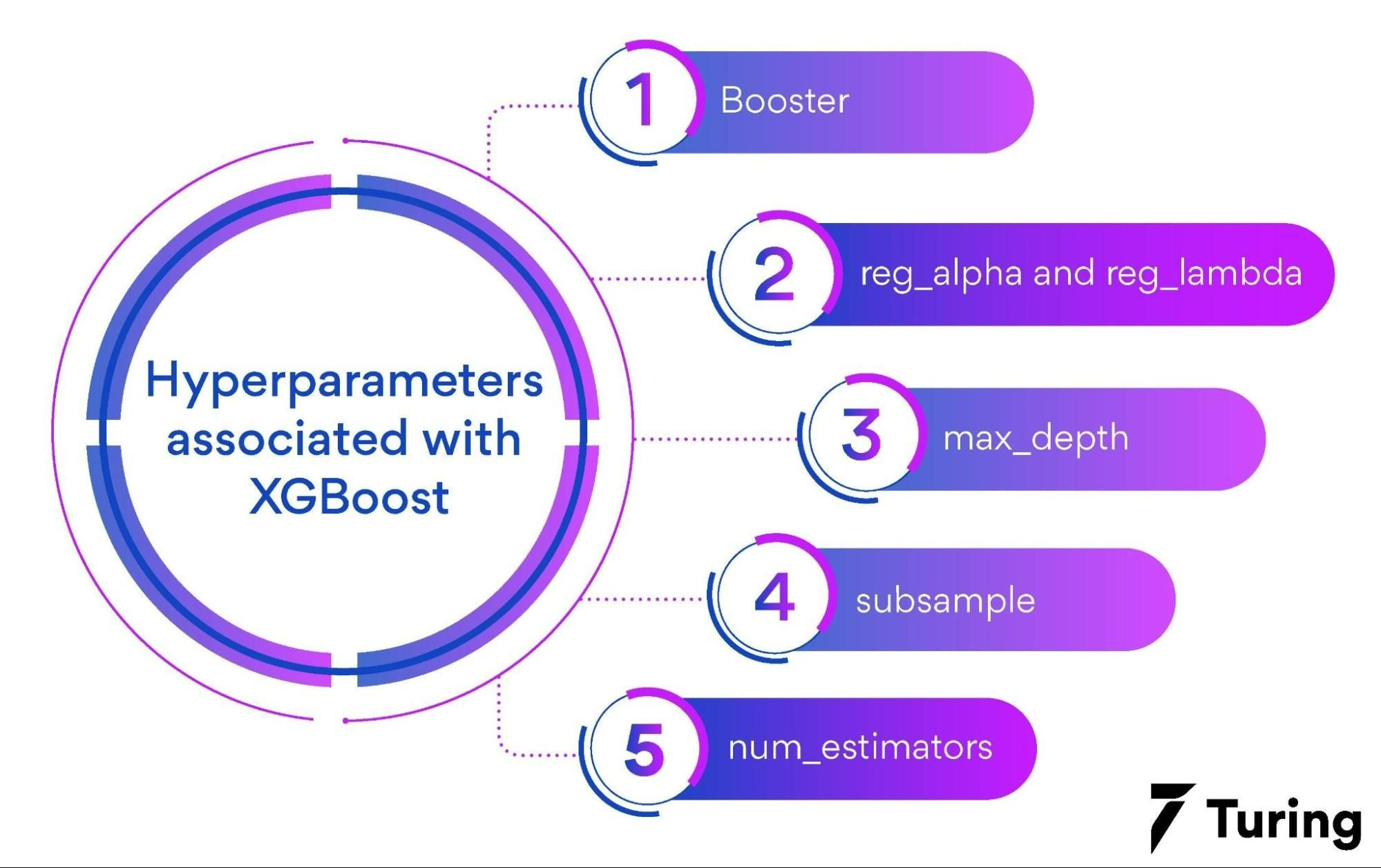Let’s understand these hyperparameters one by one.

#### Booster

Booster is a boosting algorithm and you have three options here:

- Dart: Dart is very much similar to gbtree and avoids overfitting by using the dropout techniques.

- Gblinear: Gblinear implements linear regression.

- Gbtree: Gbtree is the option by default and the gradient descent of the tree type. It will raise a penalty for facing any complexity.

#### reg_alpha and reg_lambda

reg_alpha is an L1 and reg_lambda is an L2 term. With the increase of these numbers, the model becomes more conservative. The recommended values lie between 0-1000 for both the terms.

#### max_depth

max_depth enables and sets the maximum depth for the decision trees. The higher this number, the less conservative the model becomes. There is no limit to the depth of the decision trees once this number is set to 0.

#### subsample

subsample is the size of the sample ratio that is used while training the predictors. The default value is 1 and with this value, we can use the entire data set. If this value is set to 0.7 then 70% of the observations are randomly sampled and can be used in each boosting loop. A subsample always prevents overfitting.

#### num_estimators

num_estimators set the number of boosting rounds. In short, it sets the number of boosted trees. The higher this number, the greater the risk of overfitting.

## Implementing XGBoost

To understand how XGBoost works and all about implementing it, let’s take a simple example to predict the exact number of ‘Titanic Survivors’ on a Kaggle competition.

First, download the data from Kaggle. We will need to import training data and important libraries.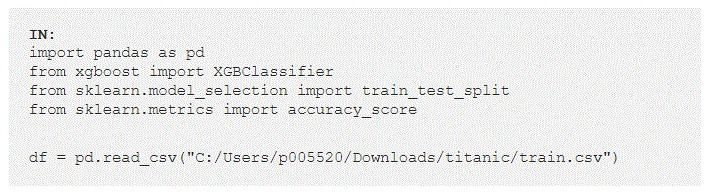In the above example, we need to note the import code ‘from xgboost import XGBClassifier’. For the code to run, we need to install XGBoost on our system by running ‘pip install xgboost’ from the terminal.

‘XGBClassifier’ is used here as we have considered a classification problem. We need to use ‘XGBRegressor’ for regression problems. The other libraries used, in the above example, process the data and help in calculating the metrics associated with model performance.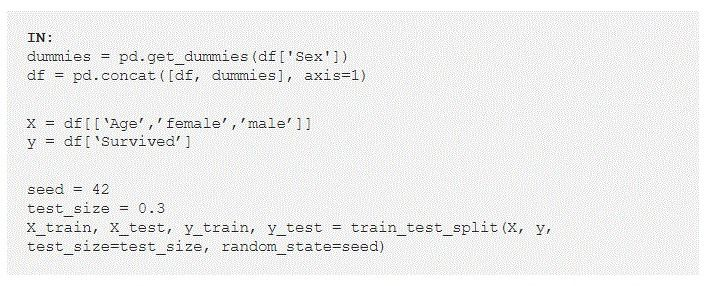Image source

We have several variables in our data and we will use the same from our previous example. We have considered the two variables: ‘age’ and ‘sex’

The first two lines of our code are used to create dummy variables from ‘sex’. This is necessary to convert the ‘sex’ variable from string to integers. These dummy variables turn into two different variables ‘male’ and ‘female’. These new variables are equal to 0 or 1 depending on the ‘sex’ of the passenger traveling on Titanic.

The next 2 lines of our code define the target variables and the variables we will use for predicting the exact number of the target variables (survivors). The remaining lines of our code are for splitting these two sets:

Test sets

The test sets are used to measure our XGBoost model performance.

Train sets

Train sets are used to create our XGBoost model.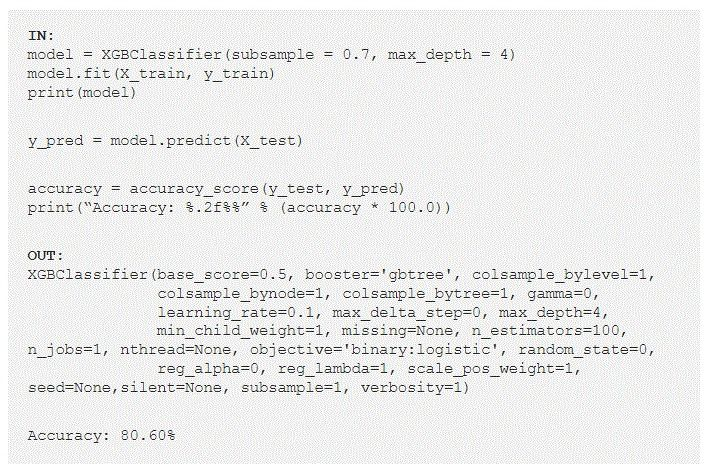Image source

We train our XGBoost model in the first two lines of our code. It should be noted that the subsample is equal to 0.7 and the maximum_depth is equal to 4. This is where we define our hyperparameters. We can see the model performance with an accuracy of 80.6% in the test set. Let’s understand how to apply the same to the new data.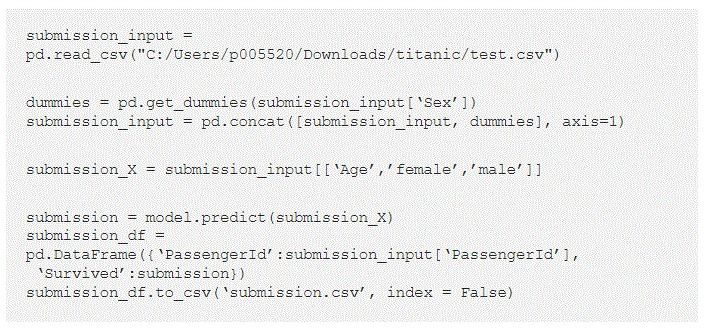Image source

In this above code fragment, we have performed a few functions.

• Imported Kaggle’s submission file

• Implemented our trained predictor with model predict (submission_X)

• Executed the same dummy treatment as we did in our training set

• Saved the file to csv

## Features of XGBoost

XGBoost is scalable in memory-limited and distributed settings too. Its efficiency to optimize with the learning algorithm makes it scalable. Here are a few features of XGBoost that make it an important element in the data science field.

### 1. Approximate algorithm

Finding the best split over a continuous feature is a hassle and to achieve this, the data needs to be stored and fitted entirely into the memory. This becomes an issue in the case of large datasets.

To avoid this problem, an approximate learning algorithm is used. The candidate split points are proposed based on the feature distribution. The continuous features are used according to the candidate split points. Post this process, the best solution is taken from the aggregated statistics.

### 2. Column block

Data sorting can be time-consuming, especially with decision trees and learning algorithms. The data is stored in the memory units called ‘blocks’ to cut down the sorting costs and also to save time. Every block has data columns that are sorted according to the feature values. Such a computation is quite sensitive and can be done only once before the training.

Block sorting can be divided between parallel threads and can be done independently with the CPU. The split finding process can be done simultaneously as the statistics of each column are collected together with the block sorting.

### 3. Weighted quantile sketch

The weighted quantile sketch is used to streamline the candidate split points and weighted datasets. This technique is used to merge the operations on quantile summaries over data.

### 4. Sparsity-aware algorithm

Some reasons such as missing values, zero entries, and one-hot encoding, scatter the input. XGBoost leverages its sparsity-aware algorithm to identify and visit the default direction in each node for a better input retrieval.

### 5. Out-of-core computation

For the data that does not fit into the main memory, XGBoost divides the data into multiple blocks and stores each memory block on the system. You can also compress blocks and decompress them on the go using an independent thread.

### 6. Regularized learning

To measure the model performance based on some parameters, we have to define the objective function. An objective function contains two elements:

• Regularization

• Training loss

The regularization term reduces the complexity of the model.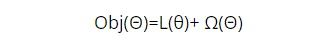Image source

In the above formula, Ω is the regularization term that has to be included in the objective functions. XGBoost includes regularization and controls the complexity of the model. Regularization learning prevents overfitting.

These 6 features described above, may present in some algorithms individually, but XGBoost combines these techniques to have an end-to-end system that improves scalability and also implements effective resource utilization.

## XGBoost attributes & benefits in data science

XGBoost offers a wide list of attributes and benefits.

• The growing data science communities and data scientists are actively contributing to developing the XGBoost further. Some benefits and features that reduce the development hours are added regularly to the open-source.

• XGBoost streamlines a wide range of applications, which includes solving problems in regression, ranking, and user-defined prediction challenges.

• XGBoost offers a huge library that is highly portable and runs on multiple platforms including Linux, OS X, and Windows.

• XGBoost also consolidates cloud integration for different ecosystems such as Yarn clusters, Azure, AWS, and many more.

• It is highly active in multiple organizations across different verticals and markets.

Although XGBoost works great with machine learning models and different learning algorithms, you should not use it as a silver bullet. For better results, you should combine feature engineering and data exploration in the field of data science. We hope this article has helped you with understanding XGBoost implementation.

## FAQs

Which algorithm is used in XGBoost?

XGBoost stands for Extreme Gradient Boosting and it deals with boosting algorithms. Using the powerful Gradient Boosting framework, it follows the distributed gradient boosting methodology in machine learning models and learning algorithms.

How does XGBoost work towards data science?

Extreme Gradient Boosting (XGBoost) is an open-source library that follows the optimized distributed gradient boosting methodology in machine learning models and learning algorithms that are optimized for modern data science problems. It streamlines the optimum utilization of the techniques associated with boosting.### Press

What's up with Turing? Get the latest news about us here.### Blog

Know more about remote work.
Checkout our blog here.### Contact

Have any questions?
We'd love to hear from you.

#### Hire and manage remote developers

Tell us the skills you need and we'll find the best developer for you in days, not weeks.